# Motion Actuation in SimMechanics R2013b

In R2013b, it is possible to specify joint motion in SimMechanics Second Generation models. In this post, I will use a simple pendulum with internal friction and subject to gravity to highlight different ways to actuate a mechanism.

If you look at the dialog of a joint like the Revolute Joint, you will notice that each primitive now has two fields in the Actuation section: Torque and Motion.

The Torque field gives you 3 options: None, Provided by Input and Automatically Computed.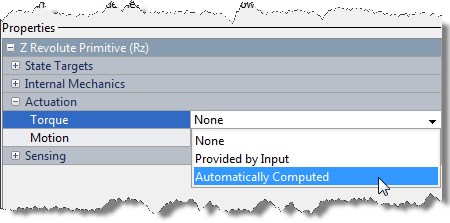The Motion field two: Provided by Input and Automatically Computed.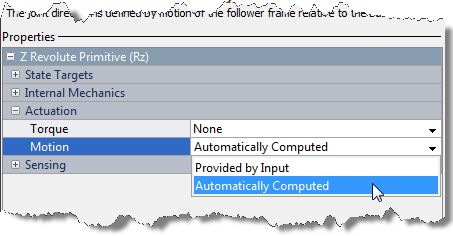This makes a total of 6 possible configurations for each joint primitive. In the Joint Actuation documentation page, you can find a description of all the modes into which a joint primitive can be actuated.

Passive: No torque and Automatically Computed Motion

This is the default setting, where the joint primitive is passive. For our example, the pendulum will simply fall under the effect of gravity.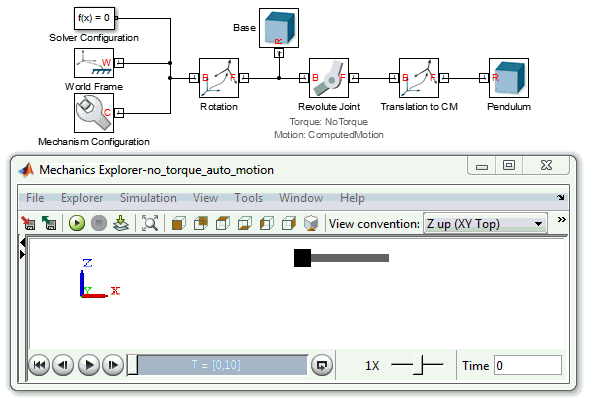Forward Dynamics: Torque Specified by Input and Automatically Computed Motion

A force or torque is specified for the joint primitive and the engine computes the resulting motion. For example, if we take the same pendulum as in the previous case and apply a constant torque, it will stabilize at an angle.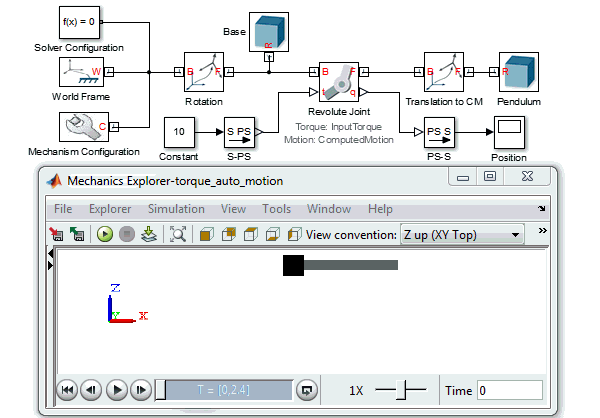Inverse Dynamics: Motion specified by Input and Automatically Computed Torque

If you know how a joint should move and want to know the amount of torque or force necessary to make that happen, this mode is for you. For example, we can apply a sine wave motion to our pendulum and measure the torque required to generate this motion: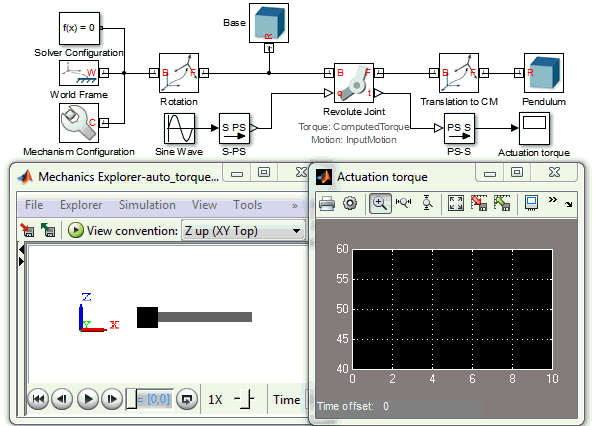One important thing to note is that when specifying motion to a joint primitive, position, velocity and acceleration must be specified. To help with that, the Simulink-PS Converter gives the possibility to filter the desired motion and internally compute the first and second derivative of the input signal.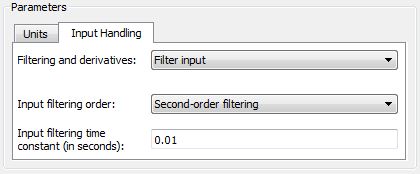***Note: By default, the time constant in the Simulink-PS Converter block is 1e-3s. This value is appropriate for most Simscape domains, but for many SimMechanics models increasing it to 1e-2s can speed up the simulation without affecting the results significantly.

If filtering is not appropriate for your application, you can always turn it off and manually provide position, velocity and acceleration to the Simulink-PS Converter.

Indirect Inverse Dynamics

This mode was not available in SimMechanics First Generation, and I decided to invent the expression indirect inverse Dynamics to describe it. As it is the case for Inverse Dynamics, the user specifies the motion of the mechanism. However the term Indirect means that SimMechanics automatically computes and applies the Forces and/or Torques necessary to produce the motion using joint primitives other than the ones where the motion is specified.

If we keep going with the same example, we can use the y-axis translational primitive of a Planar Joint to specify the translational motion of the pendulum extremity. SimMechanics can then compute the torque necessary at the pendulum base to generate this motion.***Note that I included a Weld Joint in the previous model. In R2013b, SimMechanics Second Generation has a constraint that every kinematic loop must contain at least one joint with no motion actuation and no computed torque.

Indirect Inverse Dynamics... plus external forces

The last combination available is an extension of the previous. In addition to specifying the motion, it is possible to add an additional disturbance force or torque.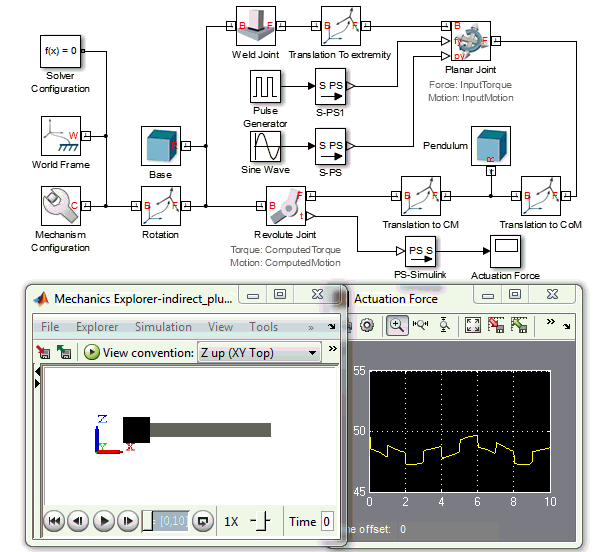Now it's your turn

Try the new motion actuation in SimMechanics R2013b and let us know what you think by leaving a comment here.

|

### Comments

To leave a comment, please click here to sign in to your MathWorks Account or create a new one.